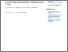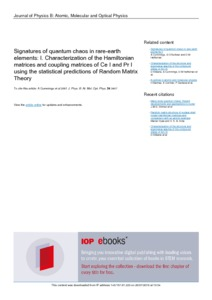# Signatures of quantum chaos in rare-earth elements: I. Characterization of the Hamiltonian matrices and coupling matrices of Ce I and Pr I using the statistical predictions of Random Matrix Theory.

Cummings, A. and O'Sullivan, G. and Heffernan, Daniel (2001) Signatures of quantum chaos in rare-earth elements: I. Characterization of the Hamiltonian matrices and coupling matrices of Ce I and Pr I using the statistical predictions of Random Matrix Theory. Journal of Physics B: Atomic, Molecular and Optical Physics, 34 (17). pp. 3407-3446.Previewmore...Add this article to your Mendeley library

## Abstract

Using the relativistic configuration interaction Hartree–Fock method the Hamiltonian matrices of Ce I, J = 4±, and Pr I, J = 11/2±, are studied. These matrices can be characterized as sparse, banded matrices, with a leading diagonal. Diagonalization of the Hamiltonian results in a set of energy eigenvalues and corresponding eigenvectors and the purpose of this investigation will be to characterize the Hamiltonian matrices and coupling matrices of Ce I and Pr I, for both ls and jj coupling representations, using various statistical predictions of Random Matrix Theory.

Item Type: Article quantum chaos; rare-earth elements; Hamiltonian matrices; coupling matrices; Ce I; Pr I; statistical predictions; Random Matrix Theory; Faculty of Science and Engineering > Mathematical Physics 9707 Prof. Daniel Heffernan 20 Jul 2018 09:57 Journal of Physics B: Atomic, Molecular and Optical Physics IOP Science YesItem control page